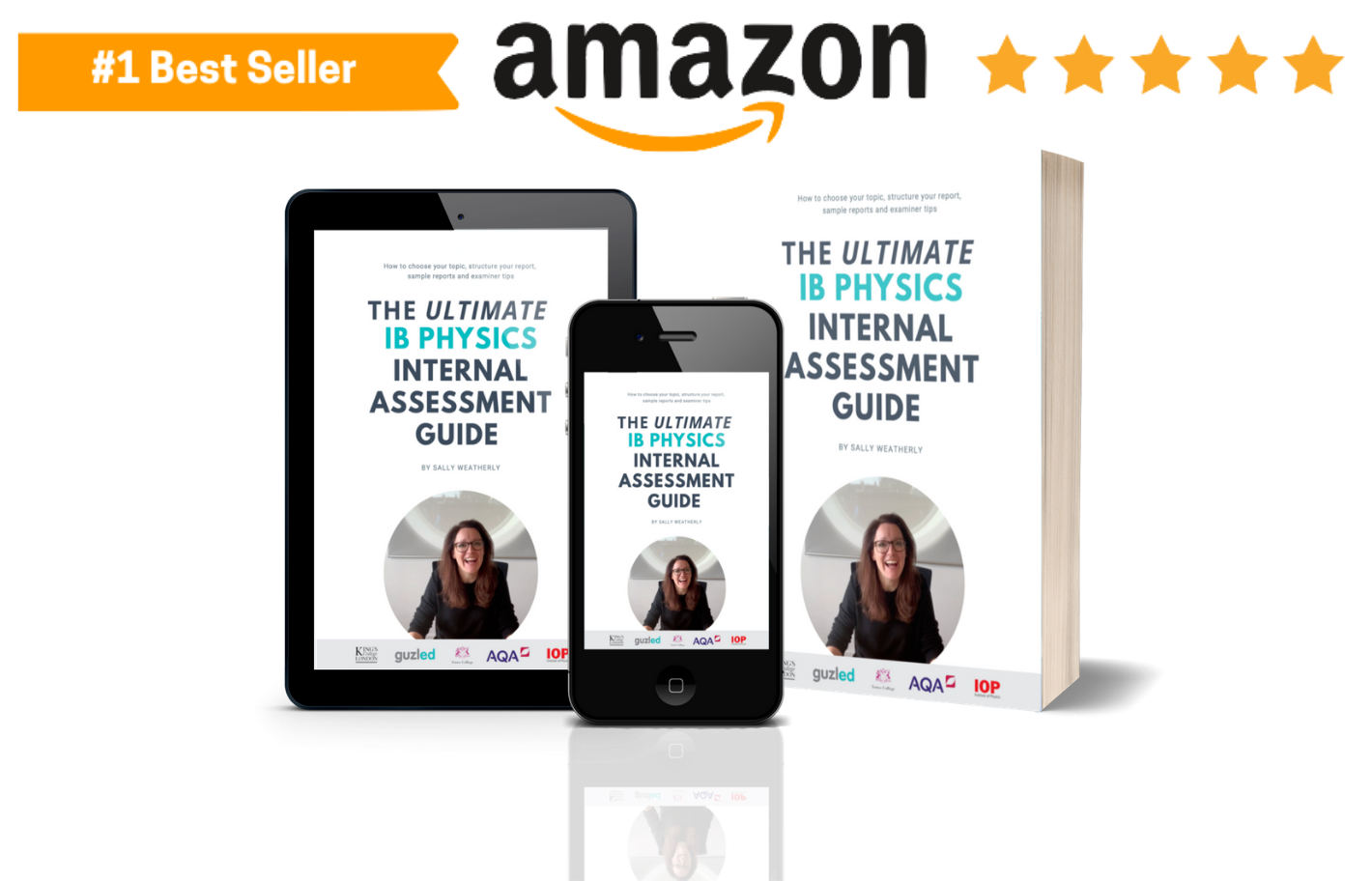# IB Physics IA | Projectile Motion (Mechanics) | Possible Research Questions

If you are considering doing your IB Physics IA on projectile Motion - it’s probably a good idea!

You can investigate it easily in a school lab and the data is easy to linearise. You just have to be careful not to make it too easy or investigate a ‘standard experiment’.

Standard experiments would be (AVOID THESE!):

• Finding the acceleration due to gravity on the Earth’s surface using projectile motion
• How does the horizontal speed of a projectile affect the range?

You should also avoid questions that will have not work! In theory, mass will have no effect on a projectile. If you are considering investigating how the mass of a projectile affects its motion - don’t!

Let me make the process of finalising a research question easy for you… CONSIDER THE DRAG EQUATION

Drag Force = Cd * A * .5 * ρ * u^2

Cd: is the drag coefficient – a dimensionless coefficient related to the projectile's geometry and taking into account both skin friction and form drag.
ρ: is the mass density of the fluid
u: is the flow velocity relative to the projectile
A: is the cross-sectional area of the projectile

So you can use the variables in this drag equation to create a really interesting (and relatively easy!) physics IA research question.

Your research question should be in the form of:

How does Variable X affect Variable Y?

Choose one of the following for your variable X*:
- cross-sectional are of projectile
- density of surrounding fluid/gas

Choose one of the following for your variable Y^:
- horizontal range
- maximum height
- time

Create a research question!

Examples of projectile motion research questions for you IB Physics IA include:
- How does the cross-sectional area of a projectile affect the horizontal range?
- How does the density of surrounding fluid of a projectile affect the time taken?
- How does the cross-sectional area of a projectile affect the maximum height?

These questions will have a relationship that is easy to linearise and will allow you lots of opportunities to expand you conclusion and evaluation of the experiment.

### FREE eBook: The ULTIMATE IB Physics Internal Assessment Guide